Technical Article

Energy Storage Using Supercapacitors: How Big is Big Enough?

December 09, 2021 by Hailey Stewart

In a power backup or holdup system, the energy storage medium can make up a significant percentage of the total bill of materials (BOM) cost, and often occupies the most volume. The key to optimizing a solution is a careful selection of components so that holdup times are met, but the system is not overdesigned.

That is, one must calculate the energy storage required to meet holdup/backup time requirements over the lifetime of the application, without excessive margin. This article presents a strategy for choosing a supercapacitor and a backup controller for a given holdup time and power, considering the vagaries of supercapacitors over their lifetimes.

Electrostatic double-layer capacitors (EDLC), or supercapacitors (supercaps), are effective energy storage devices that bridge the functionality gap between larger and heavier battery-based systems and bulk capacitors. Supercaps can tolerate significantly more rapid charge and discharge cycles than rechargeable batteries can. This makes supercaps better than batteries for short-term energy storage in relatively low energy backup power systems, short duration charging, buffer peak load currents, and energy recovery systems (see Table 1). There are existing battery-supercap hybrid systems, where the high current and short duration power capabilities of supercapacitors complement the long duration, compact energy storage capabilities of batteries.

 Feature Supercapacitors Li-Ion Battery Charge/Discharge Time <1 s to >10 s 30 min to 600 min Termination/Overcharge — Yes Charge/Discharge Efficiency 85% to 98% 70% to 85% Cycle Life 100,000+ 500+ Min to Max Cell Voltage (V) 0 to 2.3* 3 to 4.2 Specific Energy (Wh/kg) 1 to 5 100 to 240 Specific Power (W/kg) 10,000+ 1000 to 3000 Temperature (°C) –40°C to +45°C* 0°C to +45°C charge* Self-Discharge Rate High Low Intrinsic Safety High Low

Table 1. Comparison Between EDLC and Li-Ion Batteries.

 LTC3110 LTC4041 LTC3350 LTC3351 LTC3355 VIN (V) 1.8 to 5.25 2.9 to 5.5 (60 V OVP) 4.5 to 35 4.5 to 35 3 to 20 Charger (VIN → VCAP 2 A buck-boost 2.5 A buck 10+ A buck controller 10+ A buck controller 1 A buck Number of Cells 2 1 to 2 1 to 4* 1 to 4* 1 Cell Balancing Yes Yes Yes Yes — VCAP (V) 0.1 to 5.5 0.8 to 5.4 1.2 to 20 1.2 to 20 0.5 to 5 DC-to-DC (VCAP→VOUT) 2 A buck-boost 2.5 A boost 10+ A boost controller 10+ A boost controller 5 A boost VOUT Range (V) 1.8 to 5.25 2.7 to 5.5 4.5 to 35 4.5 to 35 2.7 to 5 PowerPath Internal FET External FET External FET External FET Separate boost Inrush Current Limiting — — — Yes — Systems Monitoring — PWR fail, PG V, I, cap, ESR V, I, cap, ESR VIN, VOUT, VCAP Package 24-lead TSSOP, 24-lead QFN 4 mm × 5 mm, 24-lead QFN 5 mm × 7 mm, 38-lead QFN 5 mm × 7 mm, 38-lead QFN 4 mm × 4 mm, 20-lead QFN

*Can be configured for more than four capacitors

Table 2. Feature Summary of Integrated Supercap Charger Solutions.

It is important to note that higher temperatures and higher cell voltages in supercaps decrease a supercap’s lifetime. It is important to ensure that the cell voltages do not exceed temperature and voltage ratings, and that these parameters remain within desired operation levels in applications where supercapacitors are stacked or when the input voltage is not well regulated (see Figure 1).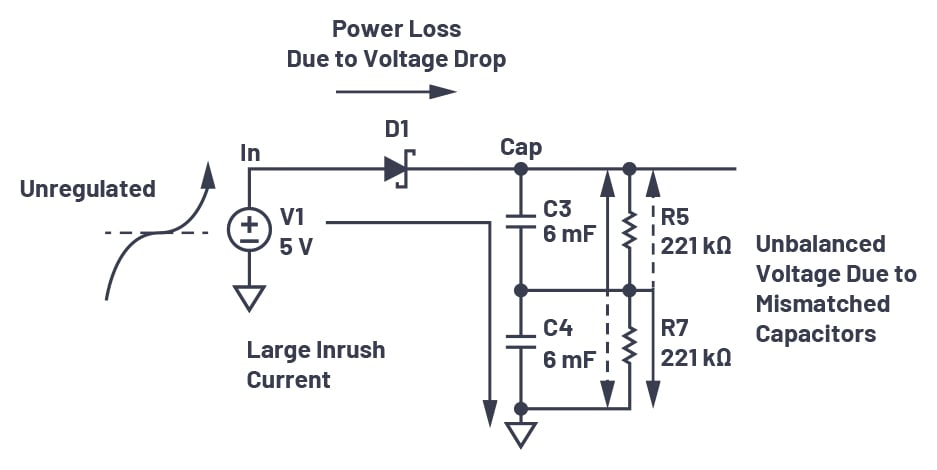Figure 1. An example of an overly simple design resulting in a risky supercap charging scheme. Image used courtesy of Bodo's Power Systems magazine.

It can be difficult to achieve a robust and efficient solution using discrete components. In contrast, integrated supercap charger/ backup controller solutions are easy to use and typically provide most or all of these features:

• A well-regulated cell voltage regardless of the input voltage variations
• Active voltage balancing of individual stacked cells to ensure the voltage is matched during all operating conditions regardless of mismatches between cells
• Low conduction losses and low dropout voltage on cell voltage to ensure the system gets the maximum amount of energy for a given supercapacitor
• Inrush current limiting for live insertion of boards
• Communication with a host controller

Selecting the Right Integrated Solution

Analog Devices has an extensive lineup of integrated solutions that incorporate all necessary circuitry to cover the fundamentals of your backup system in a single IC. Table 2 summarizes the features of some Analog Devices supercap chargers.

For applications with 3.3 V or 5 V supply rails, consider:

• The LTC3110: a 2 A bidirectional buck-boost dc-to-dc regulator and charger/balancer
• The LTC4041: a 2.5 A supercapacitor backup power manager

For applications with 12 V or 24 V supply rails, or if you require backup power beyond 10 W, consider:

• The LTC3350: a high current supercapacitor backup controller and system monitor
• The LTC3351: a hot swappable supercapacitor charger, backup controller, and system monitor

If your system requires a main buck regulator for 3.3 V or 5 V rails with a built-in boost converter for backup using a single supercapacitor or other energy source for temporary backup or ridethrough, you should consider:

• The LTC3355: a 20 V, 1 A buck dc-to-dc with integrated supercapacitor charger and backup regulator

Analog Devices also has many other constant current/constant voltage (CC/CV) solutions that can be used to charge a single supercapacitor, electrolytic capacitor, Li-Ion battery, or NiMH battery.

Calculating Holdup or Backup Time

When designing a supercapacitor energy storage solution, how big is big enough? To limit the scope of this analysis, let’s focus on the classic holdup/backup applications used in high end consumer electronics, portable industrial equipment, energy metering, and military applications.

A good analogy for this design task would be a hiker who wants to determine how much water to carry on a day-long hike. Less water at the start certainly makes going uphill easy, but he may run out of water too early, especially for a difficult hike. On the other hand, a hiker carrying a large bottle of water must endure the additional weight, but will likely stay hydrated throughout the duration of the trip. The hiker may also have to take weather into account: more water on a hot day, less when cool.

Choosing a supercapacitor is very similar; holdup duration and load are important, as is ambient temperature. Furthermore, one must take into account the lifetime degradation of the nominal capacitance and the inherent ESR of the supercapacitor. Generally, the definition of the end-of-life (EOL) parameters for supercapacitors are:

• Specified (initial) capacitance has decreased to 70% of nominal.
• ESR has doubled from the specified initial value.

These two parameters are important to the following calculations.

To size your power components, it is important to understand your holdup/ backup load specifications. In the case of a power failure, for example, the system might disable noncritical loads, so that energy can be shuttled to key circuits, such as those that save data from volatile to nonvolatile memory.

Power failures come in many forms, but generally, backup/holdup power must enable the system to gracefully shutdown in the face of a persistent failure or continue to operate through a transitory power failure.

In either of these cases, the component sizing must be worked out based on the sum of the loads that requires support during backup/holdup and the time those loads must be supported.

The amount of energy that is required to holdup or backup the system:

$Energy_{Required}=\frac{1}{Efficiency}\times Power\times Time$

The stored energy in a capacitor:

$Energy_{Stored}=\frac{1}{2}C\,V^{2}_{Capacitor}$

Common sense design dictates that the energy stored in the capacitor must be greater than what is required for holdup or backup:

$Energy_{Stored}>Energy_{Required}$

This approximates the size of the capacitor, but is not sufficient to determine the size for a truly robust system. Key details must be determined, such as the various sources of energy loss, which ultimately translate to greater required capacitance. Energy losses fall into two categories: those due to dc-to-dc converter efficiency, and those from the capacitor itself.

The efficiency of the dc-to-dc converter must be known for the condition where the supercapacitor is powering the load during holdup or backup. Efficiency depends on the duty cycle (line and load) conditions and can be obtained from the controller datasheet. The devices noted in Table 2 above have a peak efficiency of 85% to 95%, which can vary over the load current and duty cycle during the holdup or backup.

Supercapacitor energy loss amounts to the energy we cannot extract from the supercapacitor. This loss is determined by the minimum input operating voltage of the dc-to-dc converter. This is dependent on the topology of the dc-to-dc converter and is called the dropout voltage. This is an important parameter to consider when comparing integrated solutions.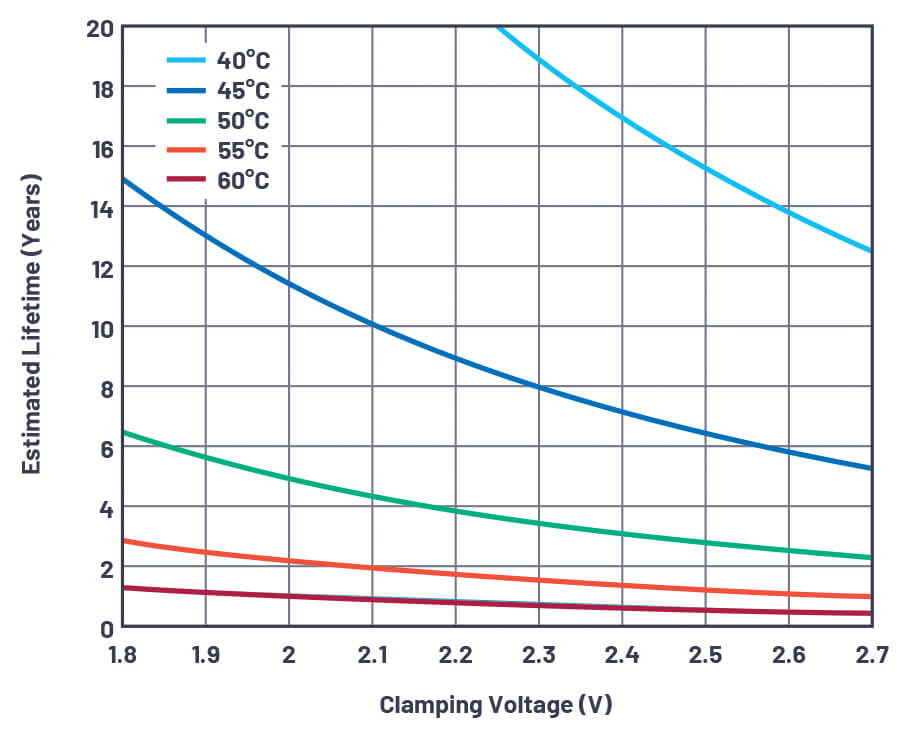Figure 2. A diagram of lifetime vs. clamping voltage, using temperature as the key parameter. Image used courtesy of Bodo's Power Systems magazine.

Taking the earlier calculation for the energy of a capacitor and subtracting the energy unavailable below VDropout results in:

What about VCapacitor? It seems obvious that setting VCapacitor to near its max rating would increase the stored energy, but this strategy has serious drawbacks. Often, supercapacitors have an absolute maximum voltage rating of 2.7 V, but the typical value is 2.5 V or less. This is due to the lifetime consideration of the application and its specified ambient temperature of operation (see Figure 2). By using a higher VCapacitor in a higher ambient temperature, the lifetime of the supercapacitor is degraded. For robust applications requiring a long operating lifetime or operation at relatively high ambient temperatures, a lower VCapacitor is best. Individual supercapacitor suppliers usually supply characteristic curves for an estimated lifetime based on clamping voltage and temperature.

Maximum Power Transfer Theorem

The third effect that must be taken into consideration is not so obvious: the maximum power transfer theorem. To obtain maximum external power from a supercapacitor source with an equivalent series resistance (see Figure 3), the resistance of the load must equal the resistance of the source. This article uses the words out, backup, or load interchangeably as all three mean the same thing in this case.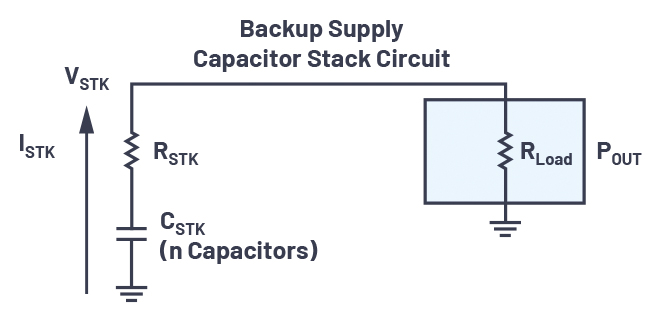Figure 3. Power delivery from a capacitor stack with series resistance. Image used courtesy of Bodo's Power Systems magazine.

If we take the diagram in Figure 3 as a Thevenin equivalent circuit, we can easily calculate the amount of power dissipated across the load via:

$P_{OUT}=V^{2}_{STK}\frac{R_{Load}}{(R_{STK}+R_{Load})^{2}}$

$P_{OUT}=(I_{STK}R_{STK})^{2}\frac{R_{Load}}{(R_{STK}+R_{Load})^{2}}$

To find the maximum power transfer, we can take the derivative of the previous equation and then solve for the condition when it is zero. This is the case when RSTK = RLOAD.

Allowing RSTK = RLOAD, we can obtain:

$P_{OUT}(MAX)=\frac{V^{2}_{STK}}{4R_{STK}}$

This can also be approached intuitively. That is, if the resistance of the load is greater than the source resistance, the load power is reduced, since the total circuit resistance goes up. Likewise, if load resistance is lower than source resistance, then most of the power is dissipated in the source due to a lower total resistance; similarly, the amount dissipated in the load is reduced. Therefore, deliverable power is maximized when source and load impedance are matched for a given capacitance voltage and a given stack resistance (ESR of the supercapacitors).Figure 4. Curve of available power vs. stack current. Image used courtesy of Bodo's Power Systems magazine.

There are implications with regard to the usable energy in a design. As the ESRs of the stacked supercapacitors are fixed, then the only value that varies during backup operation is the stack voltage and, of course, the stack current.

To satisfy the backup load requirements, as the stack voltage decreases, the required current to support the load increases. Unfortunately, increasing currents beyond the defined optimum level reduces the available backup power, as it increases the losses in the ESR of the supercapacitors. If this effect occurs before the dc-to-dc converter reaches its minimum input voltage, it translates into additional loss of usable energy.Figure 5. This diagram shows the derivation of minimum VIN required for certain output power. Image used courtesy of Bodo's Power Systems magazine.

Figure 5 shows the available power as a function of VSTK, assuming an optimal resistance matching to the load, and the graph of 25 W of backup power. This graph can also be viewed as a unitless time base: as the supercapacitors satisfy the 25 W of required backup power, the stack voltage decreases as it discharges into the load. At 3 V, there is an inflection point at which the load current is beyond the optimum level, decreasing the available backup power for the load. This is the maximum deliverable power point of the system, and at this point, losses in the ESR of the supercapacitors increase. In this example, 3 V is significantly higher than the dropout voltPower Management Figure 3: Power delivery from a capacitor stack with series resistance. Figure 4: Curve of available power vs. stack current. Figure 5: This diagram shows the derivation of minimum VIN required for certain output power. Bodo´s Power Systems® · bodospower.com 42 August 2021 age of the dc-to-dc converter, so unusable energy is due entirely to the supercapacitor, leaving the regulator underutilized. Ideally, the supercap reaches the dropout voltage, so the system’s ability to provide power is maximized.

Taking the earlier equation for PBACKUP, we can solve for VSTK(MIN). Likewise, we can also take into consideration the efficiency of the boost converter and add it to this equation:

$V_{STK(MIN)}=\sqrt{4R_{STK}P_{Backup}}$

$Boost Operation: V_{STK(MIN)}=\sqrt{\frac{4R_{STK}P_{Backup}}{\eta}}$

With this lower limit VSTK(MIN), we can establish a capacitor utilization ratio αB, which is derived from the maximum and minimum cell voltage:

$a_{B}=\frac{V^{2}_{STK(MAX)}}{V^{2}_{STK(MAX)}}$

Not only is the supercapacitor capacitance vital for determining the backup time, but the ESR of the capacitor is as well. The supercapacitor’s ESR determines how much of the stack voltage can be used for the backup load, also known as utilization ratio. As the backup process is a dynamic process in terms of input voltage, output current, and duty cycle, the complete formula for required stack capacitance is not as simple as the earlier versions. It can be shown that the final formula is:

$C_{SC}\geq\frac{2P_{Backup}t_{Backup}}{n\,\eta\,V^{2}_{TSK(MAX)}\Bigg[\frac{a_{B}+\sqrt{a_{B}}}{2}-\frac{1-a_{B}}{2}ln\Bigg(\frac{1+\sqrt{a_{B}}}{\sqrt{1-a{B}}}\Bigg)\Bigg]}$

where η = Efficiency of the dc-to-dc converter.

The concepts and calculations to this point can be translated into a supercap backup system design methodology:

• Determine the backup requirements for PBackup and tBackup.

• Determine the maximum cell voltage, VSTK(MAX), for desired lifetime of capacitor.

• Choose the number of capacitors in the stack (n).

• Choose a desired utilization ratio, αB for the supercapacitor (for example, 80% to 90%).

• Solve for capacitance CSC:

$C_{SC}\geq\frac{2P_{Backup}t_{Backup}}{n\,\eta\,V^{2}_{TSK(MAX)}\Bigg[\frac{a_{B}+\sqrt{a_{B}}}{2}-\frac{1-a_{B}}{2}ln\Bigg(\frac{1+\sqrt{a_{B}}}{\sqrt{1-a{B}}}\Bigg)\Bigg]}$

Find a supercapacitor with sufficient CSC and check if the minimum RSC formula is fulfilled:

$R_{SC}\leq\frac{\eta(1-a_{B})n\,V_{STK(MAX)}}{4P_{Backup}}$

If a suitable capacitor is not available, iterate by choosing more capacitance, a higher cell voltage, more capacitors in the stack, or a lower utilization ratio.

Taking Supercapacitor End of Life into Account

For a system that must reach a certain lifetime, the previously described methodology must be modified with EOL values, generally 70% of CNOM and 200% of ESRNOM. This complicates the math, but existing spreadsheet tools are available on product webpages for most ADI supercapacitor managers.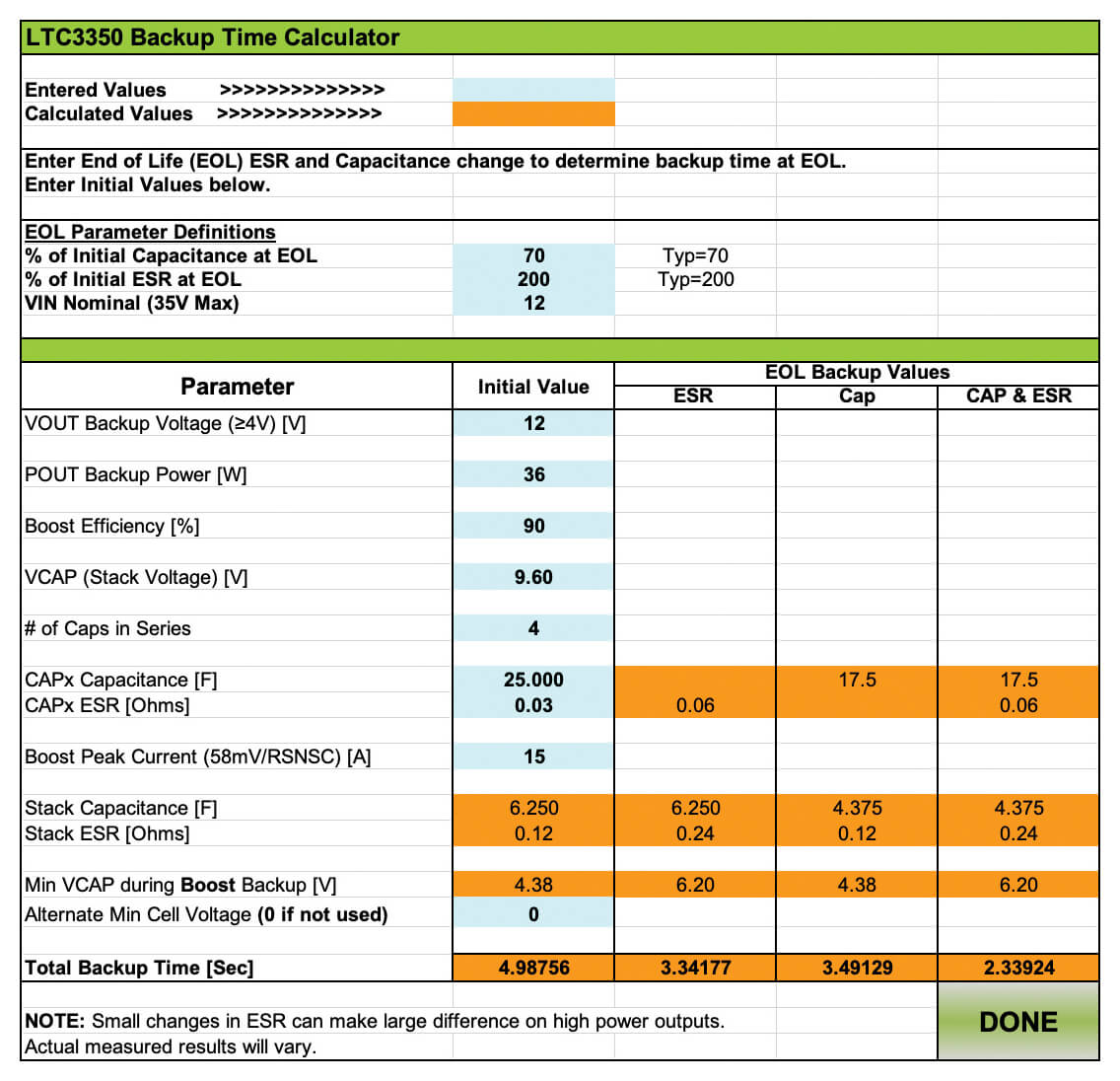Figure 6. LTC3350/LTC3351 calculation for a 36 W, 4 s holdup system with 25 F capacitance. Image used courtesy of Bodo's Power Systems magazine.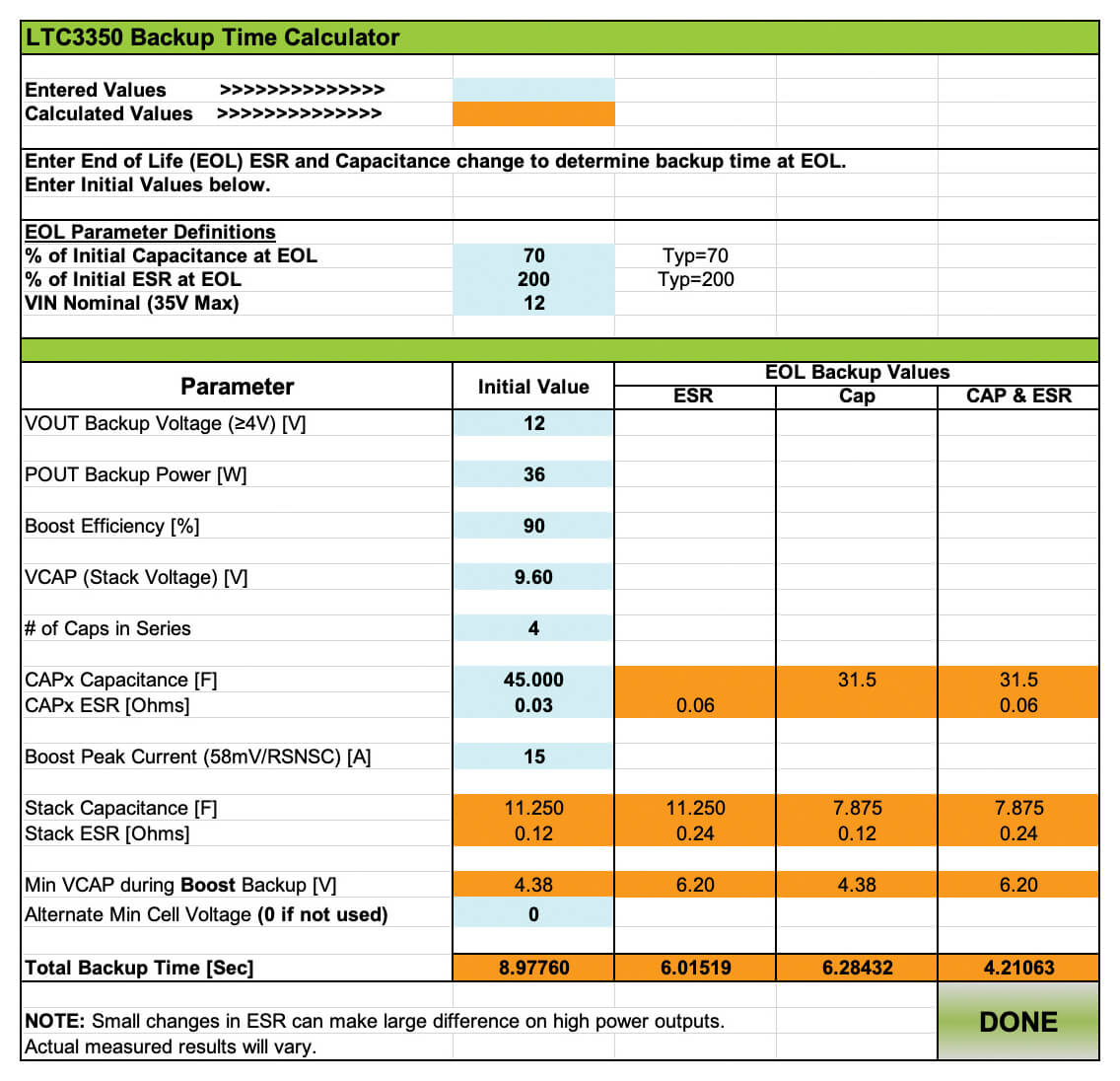Figure 7. LTC3350/ LTC3351 calculation with 45 F capacitance. Image used courtesy of Bodo's Power Systems magazine.

Let’s use a simplified methodology with example using the LTC3350:

• Required backup power is 36 W for a duration of four seconds.
• VCELL(MAX) is set to 2.4 V for longer lifetime/higher ambient temperature.
• Four capacitors are series stacked.
• DC-to-DC efficiency (ŋ) is 90%.

Using an initial guess of 25 F capacitance, the spreadsheet tool provides the result shown in Figure 6.

Based on the initial guess of 25 F capacitance, we obtain the required four seconds of backup time (with an additional 25% margin) using nominal values. However, if we consider the EOL values of ESR and capacitance, our backup time drops to almost half. To obtain four seconds with the EOL values of the capacitors, we must modify at least one of our input parameters. Since most of them are fixed, the capacitance is the most convenient parameter to increase.

• Increasing the capacitance to 45 F, the spreadsheet tool provides the result shown in Figure 7.

The necessary increase toward 45 F seems large since the nominal values provide a comfortable nine seconds of backup. However, with the addition of CAPEOL and ESREOL, and the resulting minimum stack voltage of 6.2 V, there is a sharp degradation to half of the backup time at EOL. Nevertheless, this meets our four second requirement for holdup time with an additional 5% margin.

The LTC3350 and LTC3351 offer additional telemetry features via an integrated ADC. These parts can measure the system voltages, currents, capacitance, and ESR of the supercapacitor stack. Capacitance and ESR measurements are performed with minimal impact to the system while it is online. Device configuration and measurements are communicated via I2C/SMBus. This enables the system processor to monitor important parameters over the life of the application, ensuring that available backup power meets the system requirements.

The LTC3350’s and the LTC3351’s capability to measure the capacitance and ESR of the supercapacitor stack in real time enables the user to reduce the clamp voltage when the capacitors are new and easily meet the backup requirements. The processor receiving the telemetry data can be programmed to implement the previously shown calculations. This would enable the system to calculate, on-the-fly, the minimum necessary clamp voltage to satisfy the backup time, considering realtime capacitance and ESR. This algorithm would further enhance the lifetime of the supercapacitor backup system, because, as shown in Figure 2, at elevated temperatures, the lifetime of the supercapacitors can be significantly increased by even a small decrease in the clamp voltage.

Lastly, the LTC3351 features a hot swap controller function for protection purpose. The hot swap controller uses back-to-back N-channel MOSFETs to provide foldback current limiting, which reduces inrush current and short circuit protection in highly available applications.

Conclusion

Calculating the capacitance values required to meet backup specifications can be approached as a simple power needed, power stored problem by using the basics of energy transfer at nominal values. Unfortunately, this simple approach falls short when you consider the impact of maximum power transfer, a capacitor’s EOL capacitance, and ESR. These factors greatly impact the available energy in a system over its lifetime. Using ADI’s integrated supercapacitor solutions and a number of available backup time calculation tools, analog engineers should have the confidence to design and build reliable supercapacitor backup/holdup solutions that meet design requirements over an application’s lifetime with minimum impact on cost.Image used courtesy of Bodo's Power Systems magazine.

Markus Holtkamp received his degree from the University of Bochum in 1993. He joined Linear Technology (now part of Analog Devices) in October 2010 as a field applications engineer (FAE) to provide technical support to customers in Central Europe. Markus’ experience includes 14 years as an IC designer (high speed and mixed-signal ASICs) in a German design house and 3.5 years at Arrow Electronics as an analog FAE. He is married with two children and is an avid sports participant.Image used courtesy of Bodo's Power Systems magazine.

Gabino Alonso is currently the director of strategic marketing for the Power by Linear™ Group. Prior to joining ADI, Gabino held various positions in marketing, engineering, operations, and education at Linear Technology, Texas Instruments, and California Polytechnic State University. He holds a Master of Science degree in electrical and computer engineering from University of California, Santa Barbara.

Featured Image used courtesy of Analog.